Next: Quasi-Random Walk Methods Up: A Review of Monte Previous: Introduction

# Derivation of the Rendering Equation

The main goal of computer graphics is to calculate the image that could be seen by a camera in a virtual world. This requires the calculation of the power reaching the camera from a given direction, i.e. through a given pixel, taking into account the optical properties of the surfaces and the lightsources in the virtual world.

The spectral properties of this power are responsible for the color sensation. In computer graphics the power is usually evaluated on a few (at least 3) representative wavelengths, then the colour sensation is determined from these samples.

Hereinafter, monochromatic light of a representative wavelength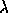will be assumed, since the complete color calculation can be broken down to these representative wavelengths. The parameters of the equations usually depend on the wavelength, but for notational simplicity, we do not always include thevariable in them.

In the next section, we briefly review the measures of the light transport and the mathematical formulation that can compute them.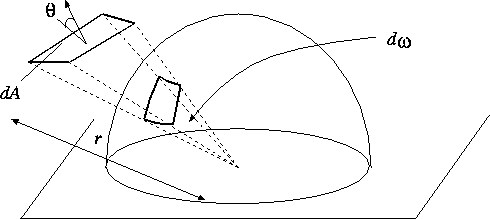Figure: Definition of the solid angle

The directional property of the energy emission is described in a so-called illumination hemisphere which contains those solid angles to where the surface point can emit energy. By definition, a solid angle is a cone or a pyramid, with its size determined by its subtended area of a unit sphere centered around the apex (figure). The solid angle, in which a differential dA surface can be seen from point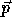, is obviously the projected area per the square of the distance of the surface. If the angle between the surface normal of dA and the directional vector from dA tois, and the distance from dA tois r, then this solid angle is: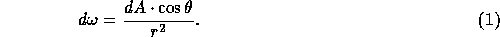The intensity of the energy transfer is characterized by several metrics in computer graphics depending on whether or not the directional and positional properties are taken into account.

The light power or flux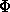is the energy radiated through a boundary per unit time over a given range of the spectrum (say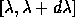).

The radiance, or intensity I, is the differential light flux leaving a surface element dA in a differential solid angle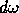per the projected area of the surface element and the size of the solid angle. If the angle of the surface normal and the direction of interest is, then the projected area is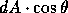, hence the intensity is: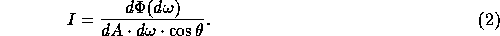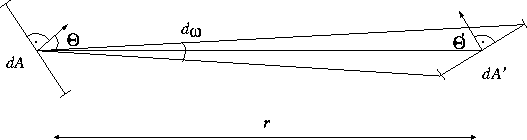Figure: Energy transfer between two differential surface elements

Having introduced the most important metrics, we turn to their determination in the simplest case, where there are only two differential surface elements in the 3D space, one (dA) emits light energy and the other (dA') absorbs it (figure). If dA' is visible from dA in solid angleand the radiant intensity of the surface element dA is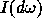in this direction, then the flux leaving dA and reaching dA' is: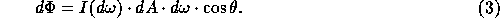according to the definition of the radiant intensity. Expressing the solid angle by the projected area of dA', we get: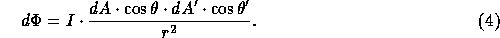This formula is called the fundamental law of photometry.

Note that the transferred power can also be written in the following form: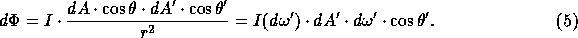Thus similar formula applies for the patch that receives the power as for the patch that emits it.

In light-surface interaction the surface illuminated by an incident beam may reflect a portion of the incoming energy in various directions or it may absorb the rest. It has to be emphasized that a physically correct model must maintain energy equilibrium, that is, the reflected and the transmitted (or absorbed) energy must be equal to the incident energy.

Optically perfect or smooth surfaces will reflect or transmit only coherent components governed by the laws of geometric optics, including the law of reflection and the Snellius-Descartes law of refraction. The incoherent components are caused by the surface irregularities reflecting or refracting the incident light in any direction. Since the exact nature of these irregularities is not known, the incoherent component is modeled by means of probability theory.

Assume that a photon comes from the direction denoted by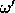to point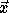. The probability of reflection or refraction atonto the solid anglearound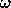is expressed by the following density function: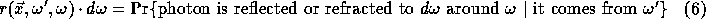Note that this probability distribution is a mixed, discrete-continuous distribution, since the probability that the light may obey the laws of geometric optics is non-zero. The energy equilibrium guarantees that the integration of the probability density over the whole sphere is 1.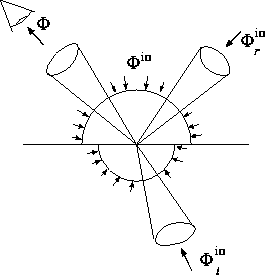Figure: Transformation of the incident light by a surface

The light flux (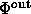) leaving the surface at the solid anglearoundconsists of the indirect and direct (emission) components.

The indirect component is expressed as the integration of the contribution of all the possible incoming directionsover the sphere around the surface: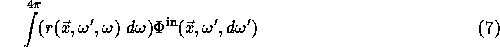If the surface itself emits energy, that is, if it is a lightsource, then the direct component (emission) also contributes to the output flux: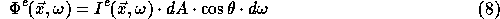Adding the possible contributions we get: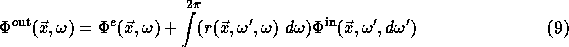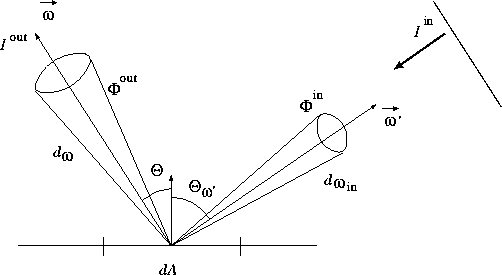Figure: Perceived Color of a surface due to incident light beams

The fluxes can be expressed by the radiant intensities, thus: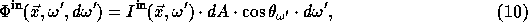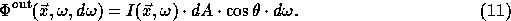Substituting these terms into equation 9 and dividing both sides by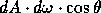we get: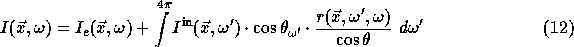Let us realize that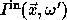is equal to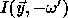if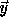is the point that is visible fromat direction, usually expressed by the so called visibility function h (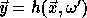).

Using these equations and introducing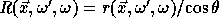that is called the bi-directional reflection/refraction function or BRDF for short, we get the following fundamental formula, called the shading, rendering or illumination equation: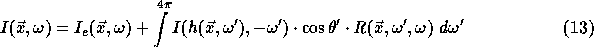Formulae of this type are called rendering equations. In fact, every color calculation problem consists of several rendering equations, one for each representative frequency. Surface parameters (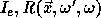) obviously vary in the different equations.

Rendering algorithms are expected to find the solution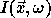of this rendering equation at least for those pointsand directions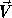which are visible from the eye at direction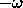. Note that the unknown intensity (also called radiance) is present both inside and outside of the integral of the equation. These types of integral equations are called Fredholm integral equations of second kind.

In order to simplify the notations, the integral operator of rendering equation is denoted by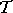: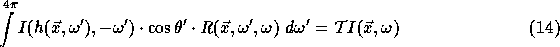Thus the short form of the rendering equation is: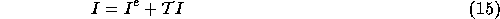There are two family of techniques that can be used to solve such an integral equation: finite element methods and random walk methods.Next: Quasi-Random Walk Methods Up: A Review of Monte Previous: Introduction

Csébfalvi Balázs
Tue Apr 15 18:39:13 METDST 1997